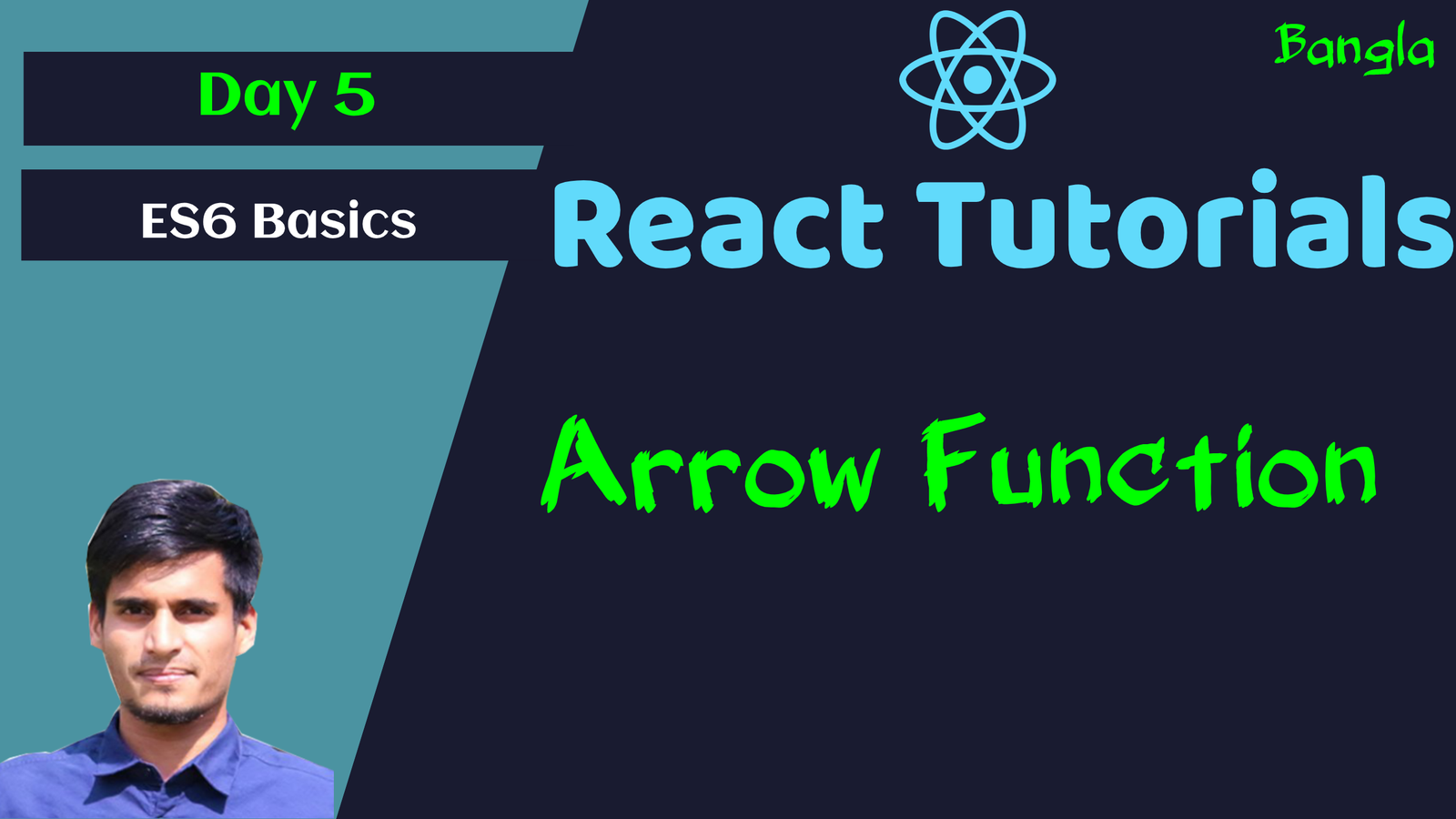Categories - React Js Tags - React Js JavaScript   Maniruzzaman Akash   3 years ago   2781   2 minutes   1

# #5 ES6 Basic - Arrow Function in Javascript - React Basic to Pro

What is function

Functions () are Statements that Do some things.

What is ES6 Arrow function

Arrow functions are ES6 short hand function syntax, lighter and smarter.

This function will get score with 20 added.

`````` const getScore = function (score) {
return score + 20;
};
``````

Now write the same code in ES6 arrow function -

``const getScore = (score) => score + 20;``

So, It's really simple and shorter. Which things are removed then in arrow function are below -

1.  Arrow function returns value by default when in one line resulting in shorter and simpler code in some cases
2. If More than one line, then it needs {} curly braces.
3. Arrow function is supported for multiple parameter also as expected.

Here is a detail example.

``````const getScore2 = () => 100;
const totalScore = (score1, score2) => {
if (score1 > 50) {
return score2;
} else {
return score1 + score2;
}
};

console.log(getScore(10));
console.log(getScore2());
console.log(totalScore(10, 1000));``````

Simple API Call Example with ES5 function and ES6 Arrow function -

``````// Traditional function
function getRepositories() {
return fetch("https://api.github.com/users/maniruzzamanakash/repos")
.then(function (response) {
return response.json();
})
.catch(function (error) {
return error;
});
}

// Arrow function
const getRepositoriesArrowFunc = () => {
return fetch("https://api.github.com/users/maniruzzamanakash/repos")
.then((response) => response.json())
.catch((error) => error);
};

console.log(getRepositories());
console.log(getRepositoriesArrowFunc());``````

So, we've see the almost same work's function is better at arrow function.

Filter Implementation in ES6 arrow function Example -

``````const arrays = [10, 20, 300, 400];
let newArray = [];
arrays.forEach(function (item) {
if (item > 100) {
newArray.push(item);
}
});
console.log(newArray);

const newArrayArrowFunc = arrays.filter((item) => item > 100);
console.log(newArrayArrowFunc);
``````

So, look at this function. In arrow function it's just one line of code and really it's very simpler, readable and optimized.

Problems:

Array function is not good with `this` keywords as before. See the code example -

``````const address = {
city: "Dhaka",
zip: 1213,
details: function () {
return `You Live in \${this.city} at zip - \${this.zip}`; // OK
},
};

city: "Dhaka",
zip: 1213,
details: function () {
return `You Live in \${this.city} at zip - \${this.zip}`; // Error
},
};